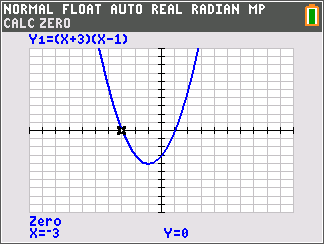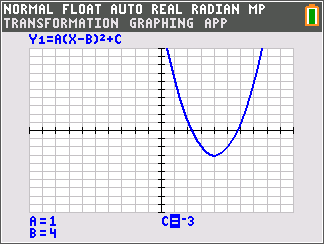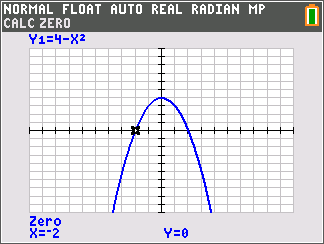# Activities

••• ##### Author9-12

45 Minutes

• ##### Device
• TI-84 Plus
• TI-84 Plus Silver Edition
•TI-84 Plus C Silver Edition
•TI-84 Plus CE
• ##### Software

TI Connect™ CE

• ##### Report an Issue#### Activity Overview

Students stretch and translate the parabola given by y = x2 and determine the effects on the equation.

#### Key Steps

•Students stretch and translate the parabola given by y = x2 and determine the effects on the equation.

•In Problem 3, students will explore a quadratic function to find the vertex and zeros of a parabola and relate them to the equation.

•In Problem 4, students will find the zeros for several given parabolas. They will try to connect the zeros with the factored form of the equation.